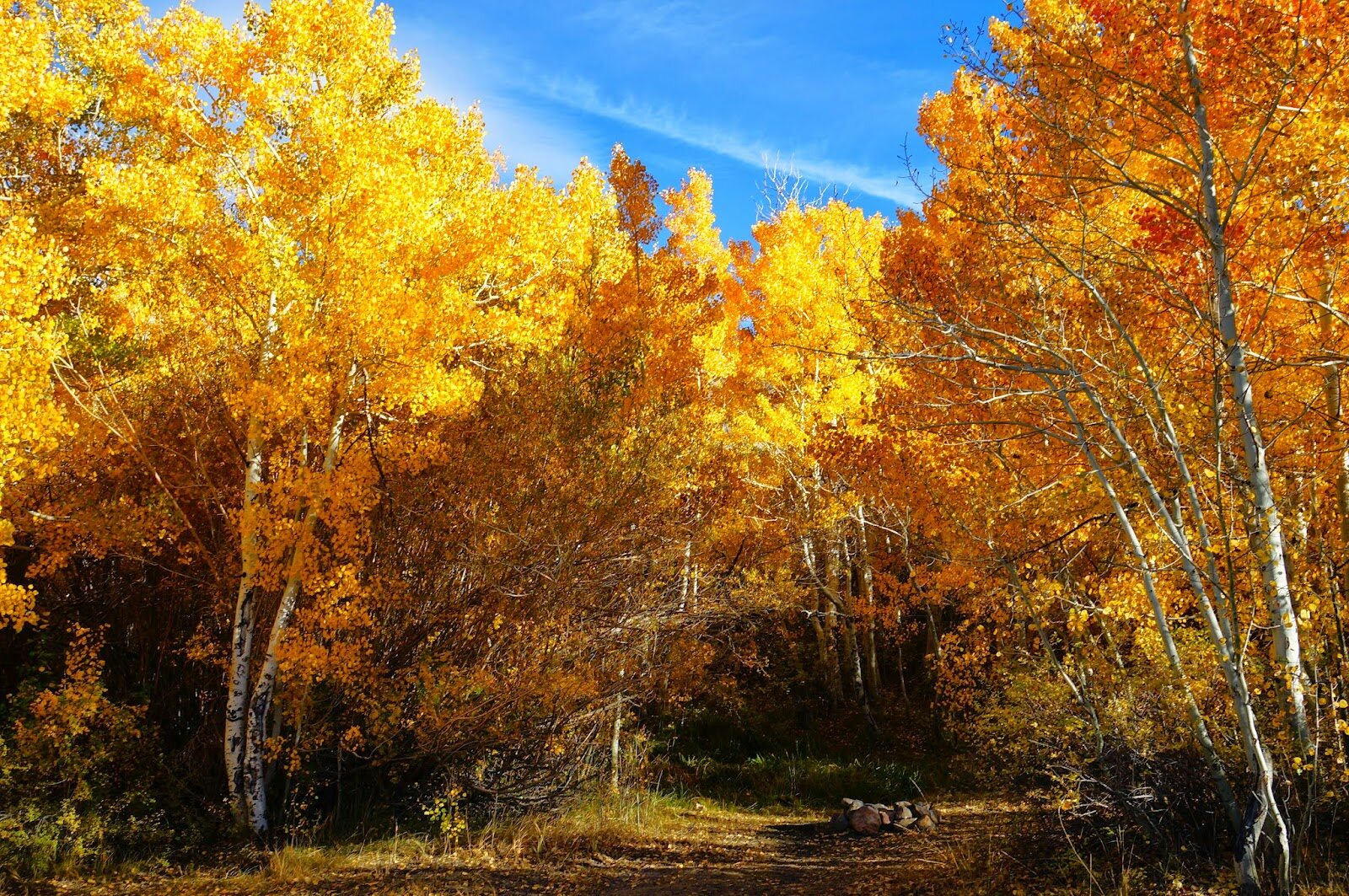# 使用 AWS EC2 上的 Apache MXNet 和 Multimedia Commons 数据集来估计图像位置### 我们的方法

Weyand 等人近期的作品提出图像定位是一个分类问题。在这种方法中，作者将地球表面细分为数千个地理单元格，并利用带地理标记的图像训练了深层神经网路。有关他们的试验更通俗的描述，请参阅该文章

col 1 col 2 col 3

    | LocationNet                                                    | PlaNet                           

S2 单元分区 | t1=5000, t2=500
→ 15,527 个单元格 | t1=10,000, t2=50
→ 26,263 个单元格

12 个训练周期 | 采用 200 个 CPU 内核时为两个半月

LocationNet 会在 MXNet Model Zoo 中公开分享。

### 下载 LocationNet

LocationNet 包括两部分：一个包含模型定义的 JSON 文件和一个包含参数的二进制文件。我们从 S3 加载必要的软件包并下载文件。

Java

import os import urllib import mxnet as mx import logging import numpy as np from skimage import io, transform from collections import namedtuple from math import radians, sin, cos, sqrt, asin path = 'https://s3.amazonaws.com/mmcommons-tutorial/models/' model_path = 'models/' if not os.path.exists(model_path): os.mkdir(model_path) urllib.urlretrieve(path+'RN101-5k500-symbol.json', model_path+'RN101-5k500-symbol.json') urllib.urlretrieve(path+'RN101-5k500-0012.params', model_path+'RN101-5k500-0012.params')

Java

# Load the pre-trained modelprefix = "models/RN101-5k500" load_epoch = 12 sym, arg_params, aux_params = mx.model.load_checkpoint(prefix, load_epoch) mod = mx.mod.Module(symbol=sym, context=mx.gpu()) mod.bind([('data', (1,3,224,224))], for_training=False)mod.set_params(arg_params, aux_params, allow_missing=True)

grids.txt 文件包含用于训练模型的地理单元格。

Java

# Download and load grids file urllib.urlretrieve('https://raw.githubusercontent.com/multimedia-berkeley/tutorials/master/grids.txt','grids.txt') # Load labels. grids = [] with open('grids.txt', 'r') as f: for line in f: line = line.strip().split('\t') lat = float(line) lng = float(line) grids.append((lat, lng))

Java

def distance(p1, p2): R = 6371 # Earth radius in km lat1, lng1, lat2, lng2 = map(radians, (p1, p1, p2, p2)) dlat = lat2 - lat1 dlng = lng2 - lng1 a = sin(dlat * 0.5) ** 2 + cos(lat1) * cos(lat2) * (sin(dlng * 0.5) ** 2)

Java

return 2 * R * asin(sqrt(a))

Java

# mean image for preprocessing mean_rgb = np.array([123.68, 116.779, 103.939]) mean_rgb = mean_rgb.reshape((3, 1, 1)) def PreprocessImage(path, show_img=False): # load image. img = io.imread(path) # We crop image from center to get size 224x224. short_side = min(img.shape[:2]) yy = int((img.shape - short_side) / 2) xx = int((img.shape - short_side) / 2) crop_img = img[yy : yy + short_side, xx : xx + short_side] resized_img = transform.resize(crop_img, (224,224)) if show_img: io.imshow(resized_img) # convert to numpy.ndarray sample = np.asarray(resized_img) * 256 # swap axes to make image from (224, 224, 3) to (3, 224, 224) sample = np.swapaxes(sample, 0, 2) sample = np.swapaxes(sample, 1, 2) # sub mean normed_img = sample - mean_rgb normed_img = normed_img.reshape((1, 3, 224, 224))return [mx.nd.array(normed_img)]

### 评估并比较模型

IM2GPS 测试集结果### 使用 URL 估算图像的地理位置

Java

Batch = namedtuple('Batch', ['data']) def predict(imgurl, prefix='images/'): download_url(imgurl, prefix) imgname = imgurl.split('/')[-1] batch = PreprocessImage(prefix + imgname, True) #predict and show top 5 results mod.forward(Batch(batch), is_train=False) prob = mod.get_outputs().asnumpy() pred = np.argsort(prob)[::-1] result = list() for i in range(5): pred_loc = grids[int(pred[i])] res = (i+1, prob[pred[i]], pred_loc) print('rank=%d, prob=%f, lat=%s, lng=%s' \ % (i+1, prob[pred[i]], pred_loc, pred_loc)) result.append(res) return result def download_url(imgurl, img_directory): if not os.path.exists(img_directory): os.mkdir(img_directory) imgname = imgurl.split('/')[-1] filepath = os.path.join(img_directory, imgname) if not os.path.exists(filepath): filepath, _ = urllib.urlretrieve(imgurl, filepath) statinfo = os.stat(filepath) print('Succesfully downloaded', imgname, statinfo.st_size, 'bytes.')

Java

return filepath

Java

#download and predict geo-location of an image of Tokyo Tower url = 'https://farm5.staticflickr.com/4275/34103081894_f7c9bfa86c_k_d.jpg'

Java

result = predict(url)

Java

rank=1, prob=0.139923, lat=35.6599344486, lng=139.728919109 rank=2, prob=0.095210, lat=35.6546613641, lng=139.745685815 rank=3, prob=0.042224, lat=35.7098435803, lng=139.810458528 rank=4, prob=0.032602, lat=35.6641725688, lng=139.746648114 rank=5, prob=0.023119, lat=35.6901996892, lng=139.692857396

### 在 Jupyter Notebook 上使用 Google Maps 直观显示结果

Java

import gmaps gmaps.configure(api_key="") # Fill in with your API key fig = gmaps.figure() for i in range(len(result)): marker = gmaps.marker_layer([result[i]], label=str(i+1)) fig.add_layer(marker) fig